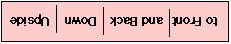# Serial Bit Order

If you look at a waveform for an asynchronous or a synchronous stream of bytes (characters) using an oscilloscope, then you'll usually notice that things are not quite as one expects. There are two things that may be unexpected:

• Since many line drivers invert the signal prior to transmission (many line receivers also invert on reception), the signal that appears on the wires can appear upside down. That is, a "1" or "idle" baud is represented by a low voltage and a "0" or "start baud" is represented as a high voltage.
• Since the start of asynchronous communications, the data bits have been sent down the wire with the least significant data bit first and the most significant bit last. Because the serialisation is usually performed by a shift register, the ordering is a result of deciding the direction of the shift.

So, the waveform observed on an oscilloscope might look like:### Asynchronous Transmission

For asynchronous transmission, the line is usually at the low voltage (OV or -12V) and that a logic 0 is sent as a high voltage (+5V or +12V). The least significant bit (lsb) of the byte/character follows the start bit (rather than the most significant), followed by the rest of the bits in the byte, the parity bit is last (if present) and then the stop baud.

Internet protocols such as ARP and Neighbor Discovery have data fields that contain link-layer (MAC) addresses. In most cases, these fields are described in "canonical form", where the first bit of each byte that appears on a cable maps to the least significant (i.e., right-most) bit of each byte in memory. Unfortunately, sometimes this is not the way that the data is presented. You need to avoid the pitfall of using non-canonical forms when canonical forms are required.

RFC 2469 provides a short note explaining this, with a useful example.

### Exception to the Rule

The bit order for all bits follows the above rules, with only one exception - that of the CRC appended to the end of some link layer frames - which is usually sent msb first.